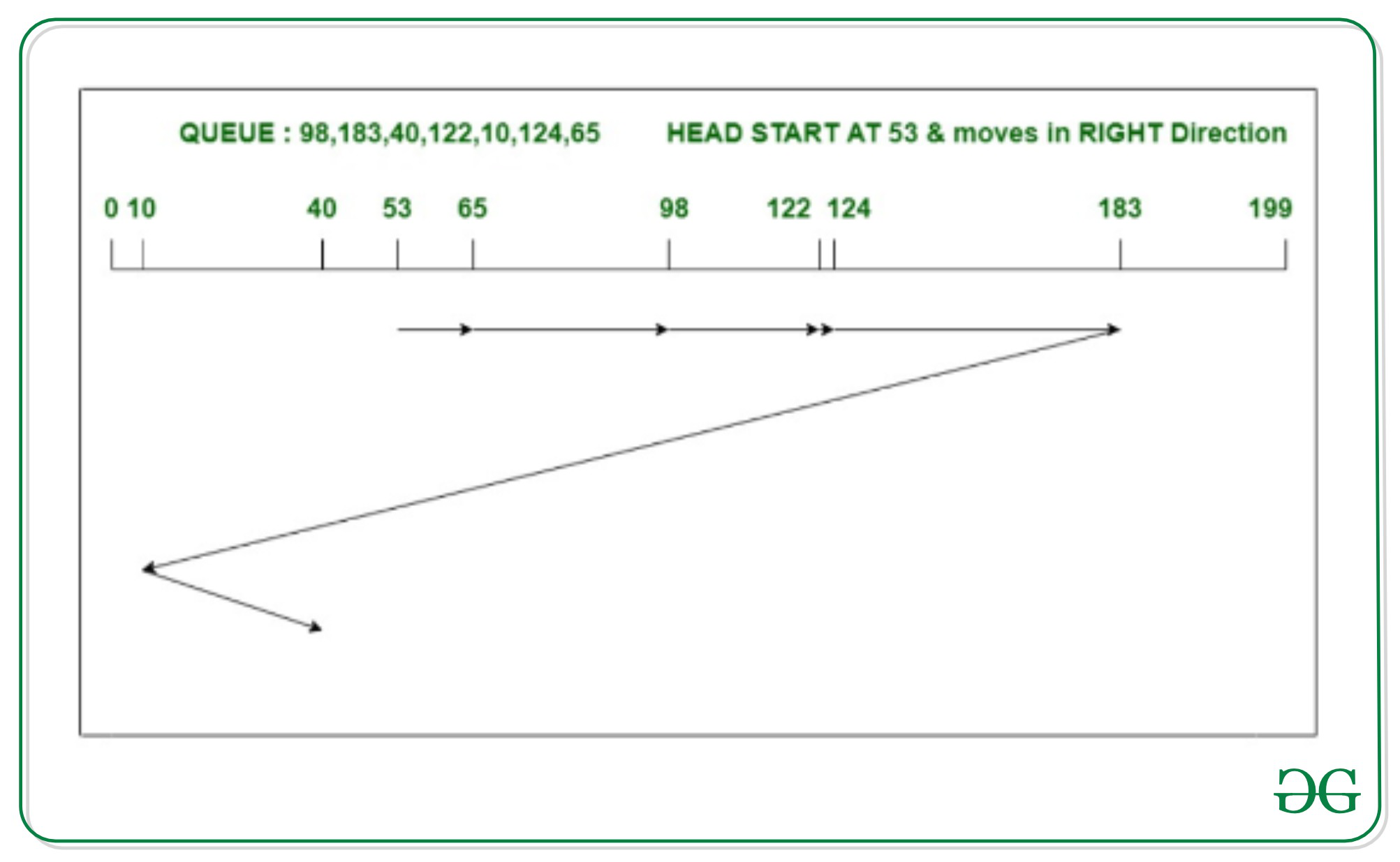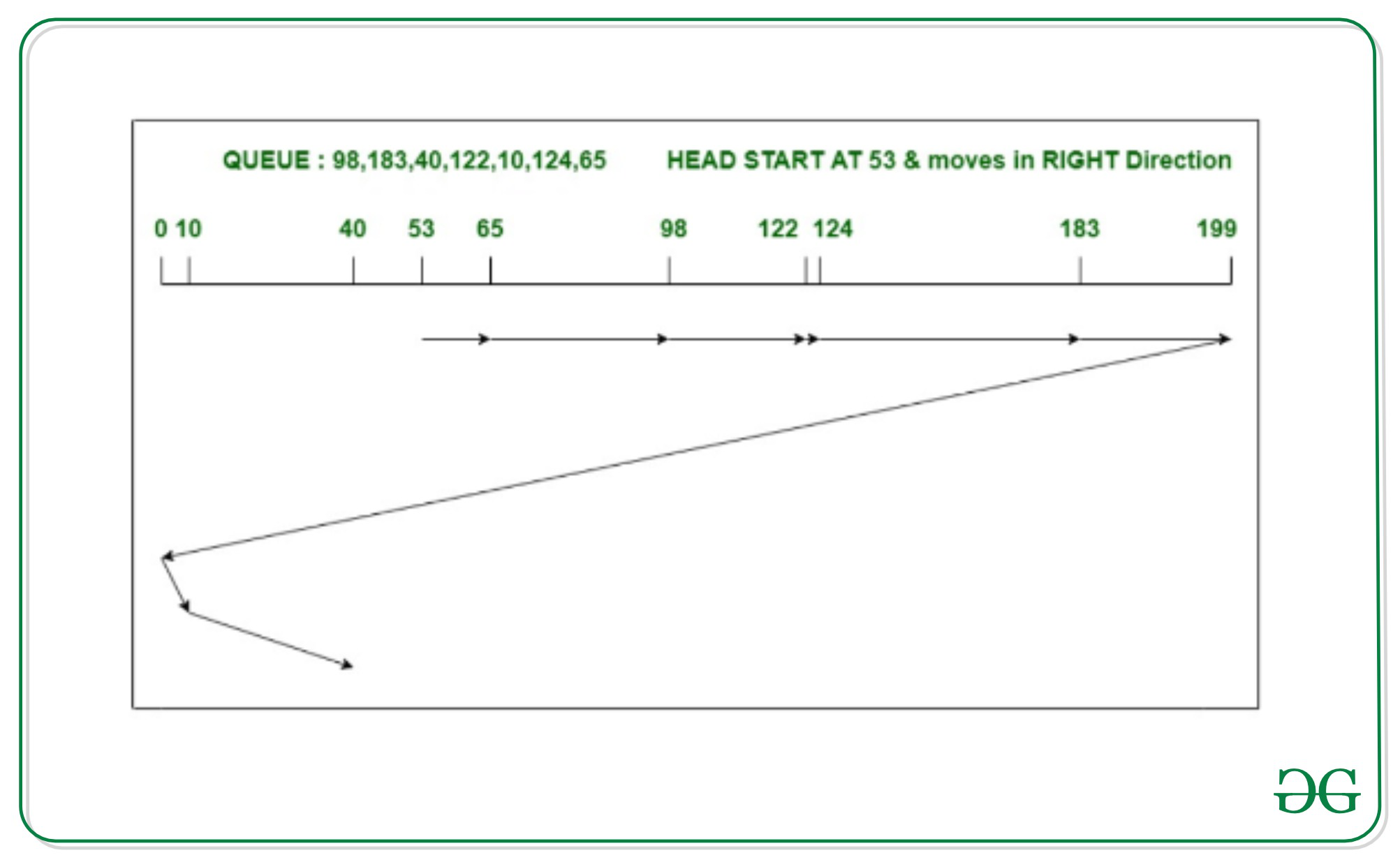Open in App
Not now

# Difference between C-LOOK and C-SCAN Disk Scheduling Algorithm

• Last Updated : 21 Jul, 2020

#### C-LOOK Disk Scheduling Algorithm

C-LOOK is the modified version of both LOOK and C-scan algorithms. In this algorithm, the head starts from the first request in one direction and moves towards the last request at another end, serving all request in between. After reaching the last request in one end, the head jumps in another direction and move towards the remaining requests and then satisfies them in the same direction as before. Unlike C-SCAN, the head pointer will move till the end request of the disk.

Example :

Consider a disk with 200 tracks (0-199) and the disk queue having I/O requests in the following order as follows:

```98, 183, 40, 122, 10, 124, 65
```

The current head position of the Read/Write head is 53 and will move in Right direction. Calculate the total number of track movements of Read/Write head using C-LOOK algorithm.```Total head movements
= (65 - 53) + (98 - 65)
+ (122 - 98)
+ (124 - 122) + (183 - 124)
+ (183 - 10) + (40 - 10)
= 333
```

#### C-SCAN Disk Scheduling Algorithm

C-scan algorithm, also known as Circular Elevator algorithm is the modified version of SCAN algorithm. In this algorithm, the head pointer starts from one end of the disk and moves towards the other end, serving all requests in between. After reaching the other end, the head reverses its direction and go to the starting point. It then satisfies the remaining requests, in the same direction as before. Unlike C-LOOK, the head pointer will move till the end of the disk, whether there is a request or not.

Example –

Consider a disk with 200 tracks (0-199) and the disk queue having I/O requests in the following order as follows:

```98, 183, 40, 122, 10, 124, 65
```

The current head position of the Read/Write head is 53 and will move in Right direction. Calculate the total number of track movements of Read/Write head using C-scan algorithm.```Total head movements
= (65 - 53) + (98 - 65)
+ (122 - 98)
+ (124 - 122) + (183 - 124)
+ (199 - 183) + (199 - 0)
+ (10 - 0) + (40 - 10)
= 395
```

### Difference between C-LOOK and C-SCAN Disk Scheduling Algorithm

My Personal Notes arrow_drop_up